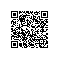# 实战项目开发细节：C语言分离一个16进制数取出相应的位1或0

其中，甄别好坏的方法是通过比如按键，或者其它的操作然后响应音频信号的输出来甄别的，那到底原理是怎么样的呢？

音频信号定制为如果是0则输出1000hz，如果是1则输出3000hz。

音频信号是一个16进制数，比如0xfd----->1111 1101

那么它的输出应该是这样的，从左边最高位开始,输出3000hz ,3000hz ,3000hz, 3000hz , 3000hz , 3000hz , 1000hz , 3000hz .

如果要求改一下，从右边最低位到最高位输出也是可以的，这个具体可以通过示波器来测量相应的信号输出波形。

原理我们搞明白了，就这么简单，于是我们就可以用C语言位运算来实现这个操作，由于公司保密特权，我不方便公开我的项目的详细细节，这样，我只能写个模拟的C语言测试程序来模仿这个过程，好了，我们开始写代码：

#include <stdio.h>
void pri() ;
void pri1() ;
int play_sound_lowtohigh(unsigned int num) ;
int bit0o1(int it,int position) ;
int play_sound_hightolow(unsigned int num) ;
int   main()
{
unsigned int num = 0xfd ; //1111 1101
play_sound_lowtohigh(num);
putchar('\n');
play_sound_hightolow(num);

return   0;
}
//从低位往高位
int play_sound_lowtohigh(unsigned int num)
{
int i   = 0;
int bit = 0 ;
for(i = 1 ; i < 9 ; i++)
{
bit = bit0o1(num,i);
if(bit == 0)
pri();
else if(bit == 1)
pri1() ;
}
}
//从高位往低位
int play_sound_hightolow(unsigned int num)
{
int i   = 0;
int bit = 0 ;
for(i = 8 ; i > 0 ; i--)
{
bit = bit0o1(num,i);
if(bit == 0)
pri();
else if(bit == 1)
pri1() ;
}
}

//it 表示数据
//position 表示第几位
int bit0o1(int it,int position)
{
int oc=1;
oc<<=(position-1);
return (oc&it)!=0?1:0;
}

void pri()
{
printf("1000hz\n") ;
}

void pri1()
{
printf("3000hz\n") ;
}#include <stdio.h>
int test_bit(int num)
{
int i ;
for(i = 7 ; i >= 0 ; i--)
{
if(num & (1 << i))
printf ("3000hz\n") ;
else
printf ("1000hz\n") ;
}
}
int main(void)
{
test_bit(0xfd) ;
}使用钉钉扫一扫加入圈子
+ 订阅